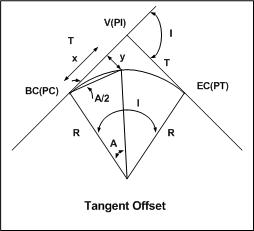* Log In to use the Calculate function * Become a Member!

Calculate The Tangent Offset Distance:

Calculation:
Designer/Checker:

Input:Radius of curve (R - ft or m)
Interior angle of arc (A - deg)

Output: